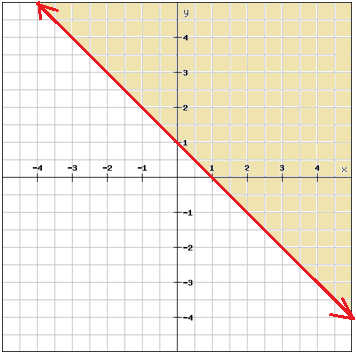# Linear inequalities in two variables

The solution of a linear inequality in two variables like Ax + By > C is an ordered pair (x, y) that produces a true statement when the values of x and y are substituted into the inequality.

Example

Is (1, 2) a solution to the inequality

$2x+3y>1$

$2\cdot 1+3\cdot 2\overset{?}{>}1$

$2+5\overset{?}{>}1$

$7>1$

The graph of an inequality in two variables is the set of points that represents all solutions to the inequality. A linear inequality divides the coordinate plane into two halves by a boundary line where one half represents the solutions of the inequality. The boundary line is dashed for > and < and solid for ≤ and ≥. The half-plane that is a solution to the inequality is usually shaded.

Example

Graph the inequality

$y\geq -x+1$## Video lesson

Graph the linear inequality

$y \geq 2x -3$